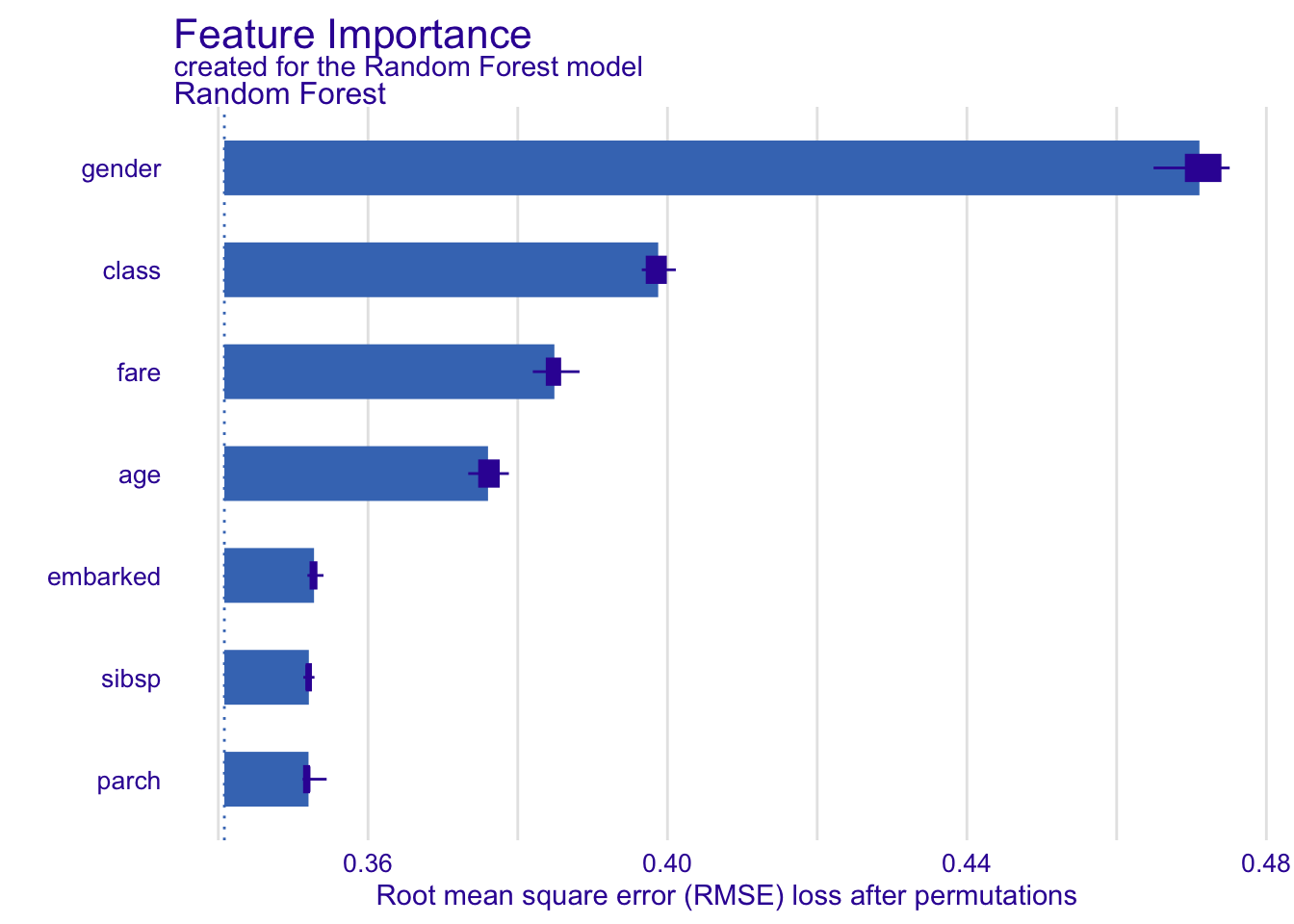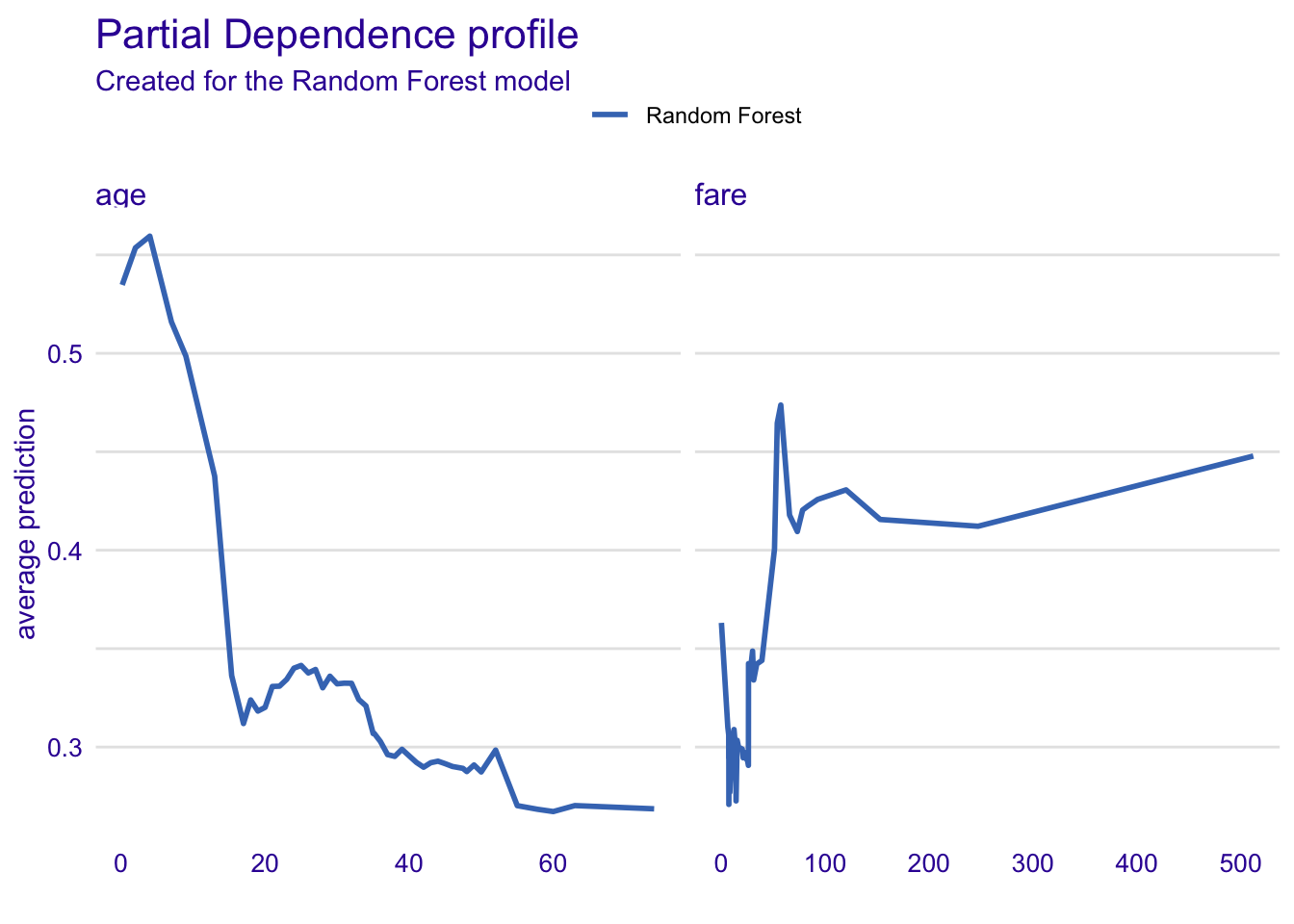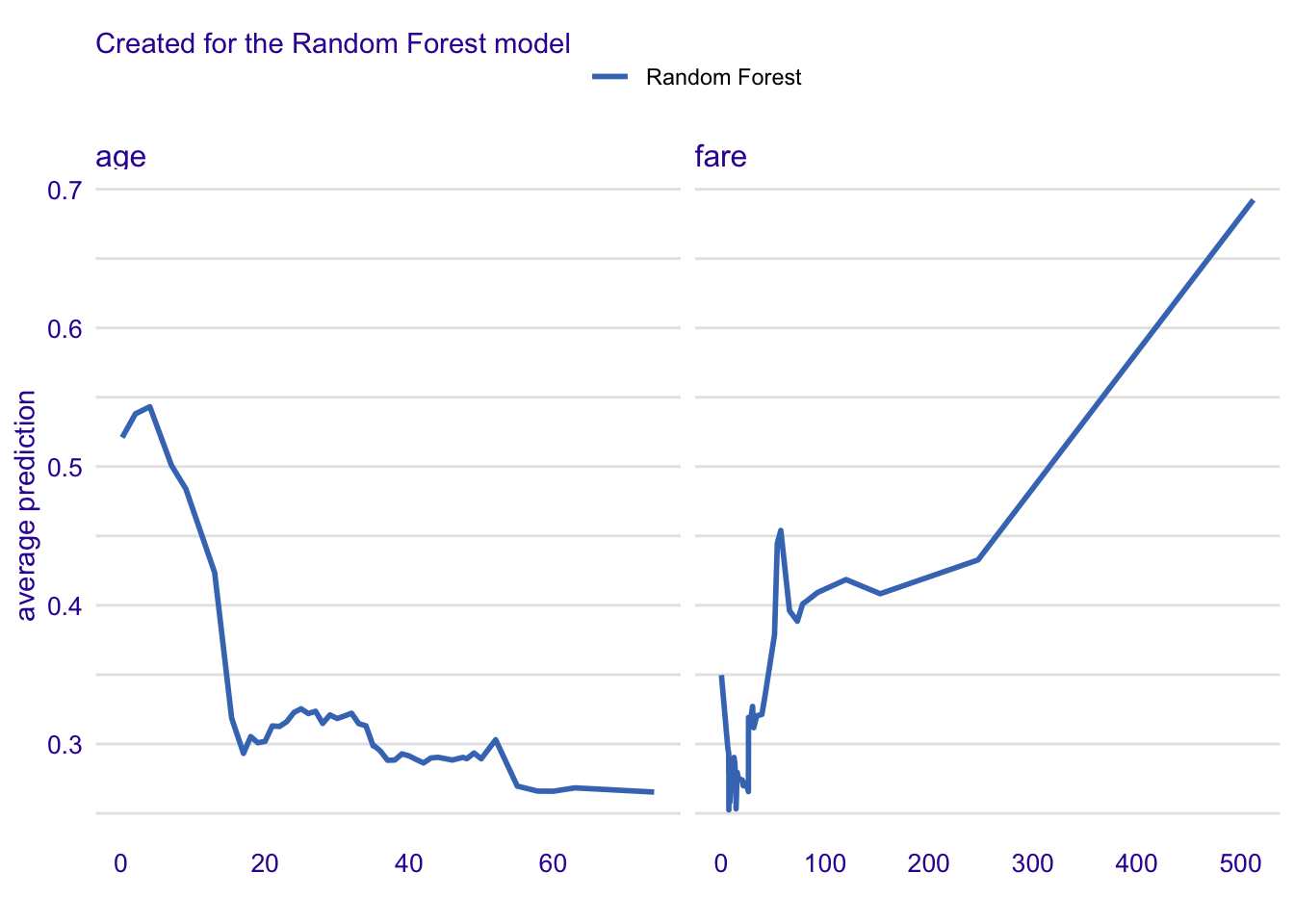# Data for Titanic survival

Let’s see an example for `DALEX` package for classification models for the survival problem for Titanic dataset. Here we are using a dataset `titanic_imputed` avaliable in the `DALEX` package. Note that this data was copied from the `stablelearner` package and changed for practicality.

``````library("DALEX")
``````#>   gender age class    embarked  fare sibsp parch survived
#> 1   male  42   3rd Southampton  7.11     0     0        0
#> 2   male  13   3rd Southampton 20.05     0     2        0
#> 3   male  16   3rd Southampton 20.05     1     1        0
#> 4 female  39   3rd Southampton 20.05     1     1        1
#> 5 female  16   3rd Southampton  7.13     0     0        1
#> 6   male  25   3rd Southampton  7.13     0     0        1``````

# Model for Titanic survival

Ok, now it’s time to create a model. Let’s use the Random Forest model.

``````# prepare model
library("ranger")
model_titanic_rf <- ranger(survived ~ gender + age + class + embarked +
fare + sibsp + parch,
data = titanic_imputed, probability = TRUE)
model_titanic_rf``````
``````#> Ranger result
#>
#> Call:
#>  ranger(survived ~ gender + age + class + embarked + fare + sibsp +      parch, data = titanic_imputed, probability = TRUE)
#>
#> Type:                             Probability estimation
#> Number of trees:                  500
#> Sample size:                      2207
#> Number of independent variables:  7
#> Mtry:                             2
#> Target node size:                 10
#> Variable importance mode:         none
#> Splitrule:                        gini
#> OOB prediction error (Brier s.):  0.1422968``````

# Explainer for Titanic survival

The third step (it’s optional but useful) is to create a `DALEX` explainer for random forest model.

``````library("DALEX")
explain_titanic_rf <- explain(model_titanic_rf,
data = titanic_imputed[,-8],
y = titanic_imputed[,8],
label = "Random Forest")``````
``````#> Preparation of a new explainer is initiated
#>   -> model label       :  Random Forest
#>   -> data              :  2207  rows  7  cols
#>   -> target variable   :  2207  values
#>   -> predict function  :  yhat.ranger  will be used (  default  )
#>   -> predicted values  :  No value for predict function target column. (  default  )
#>   -> model_info        :  package ranger , ver. 0.14.1 , task classification (  default  )
#>   -> predicted values  :  numerical, min =  0.01164526 , mean =  0.3215481 , max =  0.9899436
#>   -> residual function :  difference between y and yhat (  default  )
#>   -> residuals         :  numerical, min =  -0.7923093 , mean =  0.0006086512 , max =  0.8905081
#>   A new explainer has been created!``````

# Model Level Feature Importance

Use the `feature_importance()` explainer to present importance of particular features. Note that `type = "difference"` normalizes dropouts, and now they all start in 0.

``````library("ingredients")

fi_rf <- feature_importance(explain_titanic_rf)
``````#>       variable mean_dropout_loss         label
#> 1 _full_model_         0.3408062 Random Forest
#> 2        parch         0.3520488 Random Forest
#> 3        sibsp         0.3520933 Random Forest
#> 4     embarked         0.3527842 Random Forest
#> 5          age         0.3760269 Random Forest
#> 6         fare         0.3848921 Random Forest``````
``plot(fi_rf)``# Feature effects

As we see the most important feature is `gender`. Next three importnat features are `class`, `age` and `fare`. Let’s see the link between model response and these features.

Such univariate relation can be calculated with `partial_dependence()`.

## age

Kids 5 years old and younger have much higher survival probability.

### Partial Dependence Profiles

``````pp_age  <- partial_dependence(explain_titanic_rf, variables =  c("age", "fare"))
``````#> Top profiles    :
#>   _vname_       _label_       _x_    _yhat_ _ids_
#> 1    fare Random Forest 0.0000000 0.3630884     0
#> 2     age Random Forest 0.1666667 0.5347603     0
#> 3     age Random Forest 2.0000000 0.5536098     0
#> 4     age Random Forest 4.0000000 0.5595259     0
#> 5    fare Random Forest 6.1793080 0.3100674     0
#> 6     age Random Forest 7.0000000 0.5159751     0``````
``plot(pp_age)``### Conditional Dependence Profiles

``````cp_age  <- conditional_dependence(explain_titanic_rf, variables =  c("age", "fare"))
plot(cp_age)``````### Accumulated Local Effect Profiles

``````ap_age  <- accumulated_dependence(explain_titanic_rf, variables =  c("age", "fare"))
plot(ap_age)``````